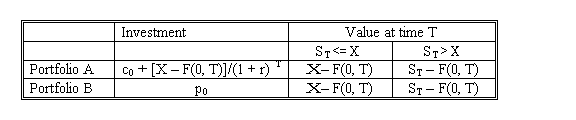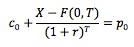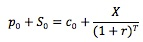### Why should I choose AnalystNotes?

AnalystNotes specializes in helping candidates pass. Period.

### Subject 11. Put-call parity for options on forwards

Assume that:

• F(0, T) is the price established today for a forward contract expiring at time T.
• c0: the call option price today.
• p0: the put option price today.
• Both options expire when the forward contract expires: the time until expiration is also T.
• The exercise price of both options is X.

Consider two portfolios. Portfolio A consists of a long call and a long position in a zero-coupon bond with face value of X - F(0, T). Portfolio B consists of a long put and a long forward.As two portfolios have exactly the same payoff, their initial investments should be the same as well. That is:This equation is put-call parity for options on forward contracts.

As F(0, T) = S0(1 + r)T, we rearrange the equation as the follows:Consider the following example:

T = 90 days, r = 5%, X = \$95, S0 = \$100, and the call price is \$10. The put price should be c0 + X/(1 + r)T - S0 = 10 + 95/(1 + 0.05) (90/365) - 100 = \$3.86.

Similarly, we can compute the call price given the price of the put.

Consider another example. The options and a forward contract expire in 50 days. The risk-free rate is 6%, and the exercise price is 90. The forward price is 92, and the call price is 5.5.

p0 = c0 + [X - F(0, T)]/(1 + r)T = 5.5 + (90 - 92)/1.06(50/365) = 3.52.

Note that in this case X < F(0, T), which means we short the bond instead of buying the bond as in portfolio A above.

Continue with those assumptions at the beginning of this subject. Consider a portfolio consisting of a long call, short put and a long position in a zero-coupon bond with face value of X - F(0, T). At expiration the value of the portfolio is:

• 0 (value of long call) + [-(X - ST)] (value of short put) + [X - F(0, T)] (value of long bond) = ST - F(0, T), if ST <= X.
• [ST - X] (value of long call) + 0 (value of short put) + [X - F(0, T)] (value of long bond) = ST - F(0, T), if ST > X.

As a forward contract's payoff at expiration is also ST - F(0, T), the portfolio's initial value must be equal to the initial value of the forward contract (which is 0).Solving for F(0, T), we obtain the equation for the forward price in terms of the call, put, and bond. Therefore, a synthetic forward contract is a combination of a long call, a short put and a zero-coupon bond with face value (X - F(0, T)). Note that we may either long or short this bond, depending on whether the exercise price of these options is lower or higher than the forward price.

#### Practice Question 1

The equation for put-call-forward parity is

A. p0 = c0 + [X - F(0, T)]/(1 + r)T
B. c0 = p0 + [X - F(0, T)]/(1 + r)T
C. p0 = c0 + [F(0, T) - X]/(1 + r)T
D. c0 = p0 - [F(0, T) - X]/(1 + r)T

#### Practice Question 2

To create a synthetic forward contract, we should

A. long a call, short a put, and long a risk-free bond.
B. short a call, long a put, and long a risk-free bond.
C. long a call, short a put, and long or short a risk-free bond.

The synthetic forward contract would have an initial value of c0 - p0 + [X - F(0, T)]/(1 + r)T. If X - F(0, T) > 0, we would long the bond; if X - F(0, T) < 0, we would short the bond. If X = F(0, T), then the bond is out of the picture.

#### Practice Question 3

The options and a forward contract expire in 90 days. The continuously compounded risk-free rate is 5%, and the exercise price is 75. The call price is 5.5, and the put price is 9.2. What is the forward price?

Rearrange the put-call-forward parity equation: F(0, T) = (c0 - p0)(1 + r)T + X = (5.5 - 9.2) 1.05 (90/365) + 75 = 71.26.

#### Practice Question 4

Consider the following information on put and call options on an asset:

Put price: p0 = 8.
Exercise price: X = 60.
Forward price: F(0, T) = 55.
Days to option expiration: 180 days.
The continuously compounded risk-free rate: r(c) = 4%.

Use put-call-forward parity to calculate the price of a synthetic call option.

c0 = long forward + p0 - [X - F(0, T)]/(1 + r)T = 0 + 8 - (60 - 55)/1.04180/365 = 3.10.

#### Practice Question 5

Consider the following information on put and call options on an asset:
Call price: 3.1.
Put price: p0 = 9.
Exercise price: X = 60.
Forward price: F(0, T) = 55.
Days to option expiration: 180 days.
The continuously compounded risk-free rate: r(c) = 4%.

To make a risk-free profit using a synthetic put, you would:

A. long call and bond, short forward and put.
B. long call and forward, short bond and put.
C. long put and forward, and short call and bond.

The present value of the bond is [X - F(0, T)] / (1 + r)T = (60 - 55) / 1.04180/365 = 4.9.
The price of a synthetic put would be: p0 = short forward + c0 + [X - F(0, T)]/(1 + r)T = 0 + 3.1 + 4.9 = 8.
As the actual put is more expensive, we should sell the put and buy the synthetic put (long call, short forward and long bond).

The initial up-front cash is generated as -3.1 (long call) - 4.9 (long bond) + 0 (short forward) + 9 = 1.
At expiration, short forward would generate - (ST - 55), and long bond would generate (60 - 55).
• If ST < 60, the portfolio would generate 0 (long call) - (ST - 55) (short forward) - (60 - ST) (short put) + (60 - 55) (long bond) = 0.
• If ST >= 60, the portfolio would generate (ST - 60) (long call) - (ST - 55) (short forward) + 0 (short put) + (60 - 55) (long bond) = 0.
The strategy would generate 1 up-front without any investment or any amount to pay back later.

#### Practice Question 6

Consider the following information on put and call options on an asset:

Call price: 2.6.
Put price: p0 = 8.
Exercise price: X = 60.
Forward price: F(0, T) = 55.
Days to option expiration: 180 days.
The continuously compounded risk-free rate: r(c) = 4%.

To make a risk-free profit using a synthetic call, you would:

A. long call and bond, short forward and put.
B. long call and forward, short bond and put.
C. long put and forward, and short call and bond.